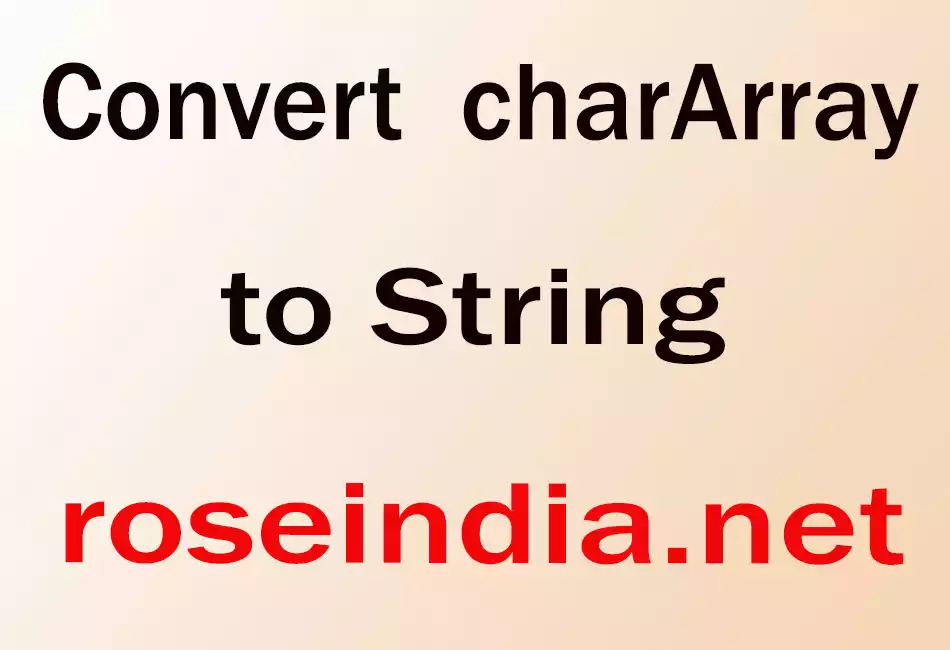# Convert charArray to String

In this section, you will learn how to convert chararray to String .### Convert charArray to String

In this section, you will learn how to convert chararray to String .# Convert charArray to String

Lets see how to convert charArray to String.

Code Description:

In this program we have taken an Array of characters as
char [] array = { '(','B','o','n','j','o','u','r',' ','m','a','d','a','m','e',')' }; and we have passed it to a String. Hence we get the following output as shown below.

Here is the code of this program:

 ``` public class charArraytoStr{   public static void main(String args[]){   char [] array = { '(','B','o','n','j','o','u','r',' ','m','a', 'd','a','m','e',')' };   String s = new String(array);   System.out.println(s);     } }```

Output of the program:

 C:\unique>javac charArraytoStr.java C:\unique>java charArraytoStr (Bonjour madame)